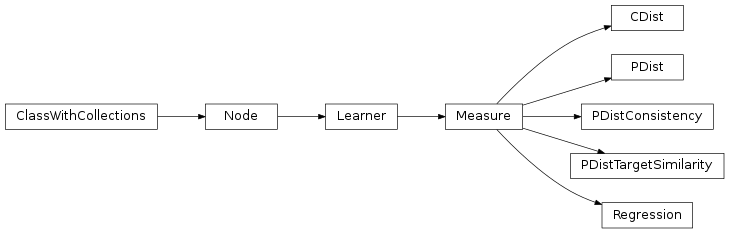# mvpa2.measures.rsa¶

Representational (dis)similarity analysisFunctions

 `cdist`(XA, XB[, metric, p, V, VI, w]) Computes distance between each pair of the two collections of inputs. `mean_group_sample`(attrs[, attrfx]) Returns a mapper that computes the mean samples of unique sample groups. `pdist`(X[, metric, p, w, V, VI]) Pairwise distances between observations in n-dimensional space. `pearsonr`(x, y) Calculates a Pearson correlation coefficient and the p-value for testing non-correlation. `rankdata`(a[, method]) Assign ranks to data, dealing with ties appropriately. `squareform`(X[, force, checks]) Converts a vector-form distance vector to a square-form distance matrix, and vice-versa.

Classes

 `CDist`(\*\*kwargs) Compute cross-validated dissimiliarity matrix for samples in a dataset `Dataset`(samples[, sa, fa, a]) Generic storage class for datasets with multiple attributes. `EnsureChoice`(\*values) Ensure an input is element of a set of possible values `Measure`([null_dist]) A measure computed from a `Dataset` `PDist`(\*\*kwargs) Compute dissimiliarity matrix for samples in a dataset `PDistConsistency`(\*\*kwargs) Calculate the correlations of PDist measures across chunks `PDistTargetSimilarity`(target_dsm, \*\*kwargs) Calculate the correlations of PDist measures with a target `Parameter`(default[, constraints, ro, index, ...]) This class shall serve as a representation of a parameter. `Regression`(predictors[, keep_pairs]) Given a dataset, compute regularized regression (Ridge or Lasso) on the computed neural dissimilarity matrix using an arbitrary number of predictors (model dissimilarity matrices). `combinations` combinations(iterable, r) –> combinations object `product` product(*iterables) –> product object